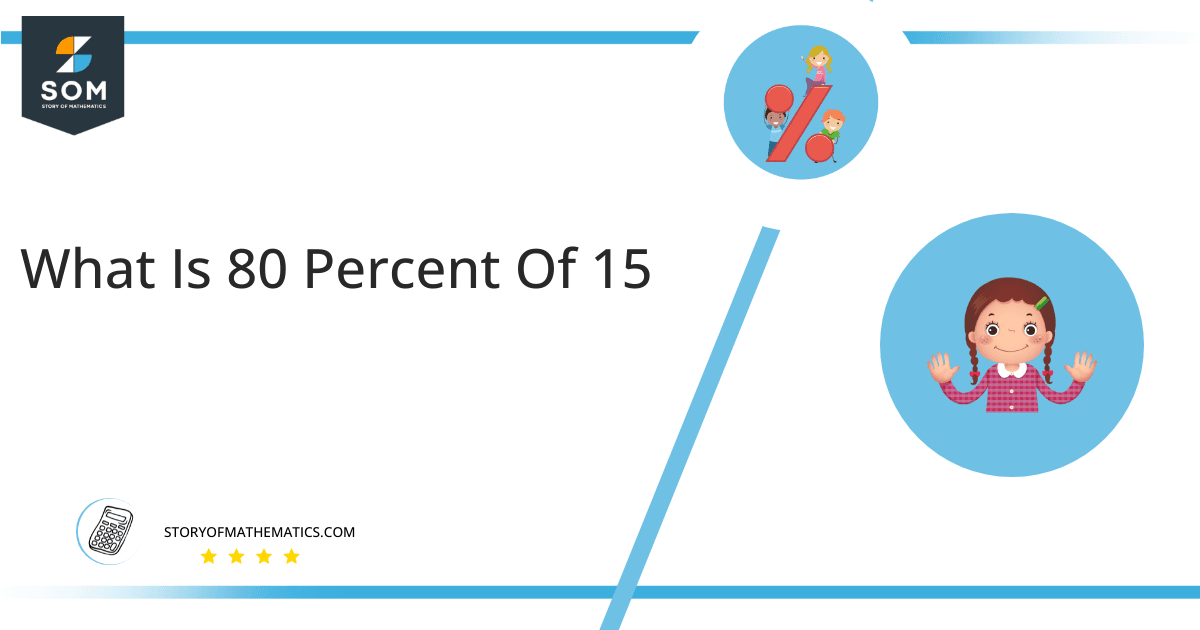# What Is 80 Percent of 15 + Solution with Free Steps?

The 80 percent of 15 is equal to 12. It can be easily calculated by dividing 80 by 100 and multiplying the answer with 15 to get 12.The easiest way to get this answer is by solving a simple mathematical problem of percentage. You need to find 80% of 15 for some sale or real-life problem. Divide 80 by 100, multiply the answer with 15, and get the 80% of 15 value in seconds.

This article will explain the full process of finding any percentage value from any given quantity or number with easy and simple steps.

## What Is 80 percent of 15?

The 80 percent of 15 is 12.

The percentage can be understood with a simple explanation. Take 15, and divide it into 100 equal parts. The 80 number of parts from the total 100 parts is called 80 percent, which is 12 in this example.

## How To Calculate 80 percent of 15?

You can find 80 percent of 15 by some simple mathematical steps explained below.### Step 1

Firstly, depict 80 percent of 15 as a fractional multiple as shown below:

80% x 15

### Step 2

The percentage sign % means percent, equivalent to the fraction of 1/100.

Substituting this value in the above formula:

= (80/100) x 15

### Step 3

Using the algebraic simplification process, we can arithmetically manipulate the above equation as follows:

= (80 x 15) / 100

= 1200 / 100

= 12This percentage can be represented on a pie chart for visualization. Let us suppose that the whole pie chart represents the 15 value. Now, we find 80 percent of 15, which is 12. The area occupied by the 12value will represent the 80 percent of the total 15 value. The remaining region of the pie chart will represent 20 percent of the total 15 value. The 100% of 15 will cover the whole pie chart as 15 is the total value.

Any given number or quantity can be represented in percentages to better understand the total quantity. The percentage can be considered a quantity that divides any number into hundred equal parts for better representation of large numbers and understanding.

Percentage scaling or normalization is a very simple and convenient method of representing numbers in relative terms. Such notations find wide application in many industrial sectors where the relative proportions are used.